# Current Electricity and Effects of Current

12. Dec 2020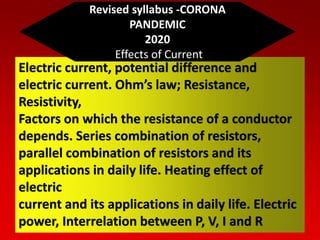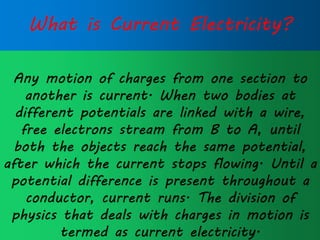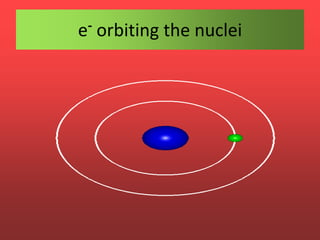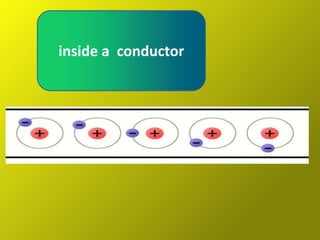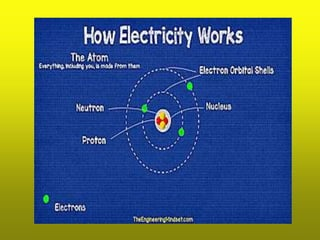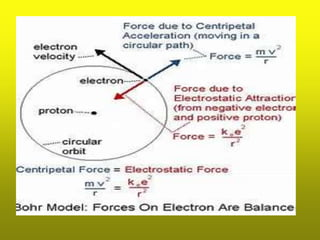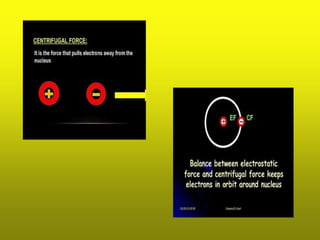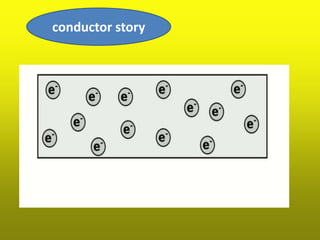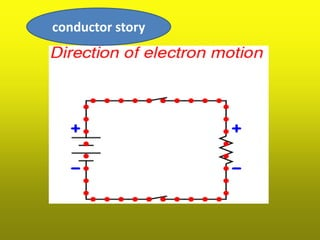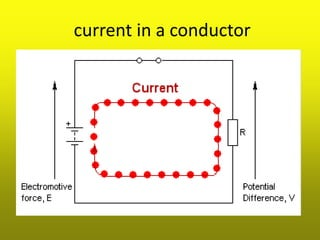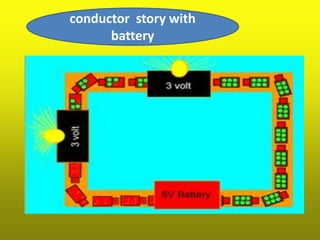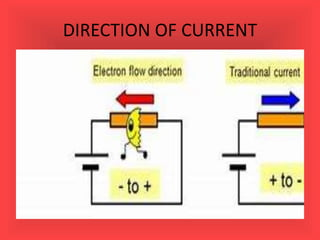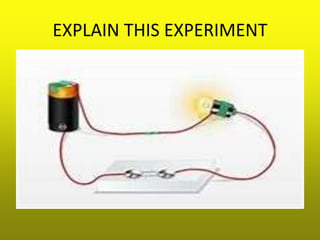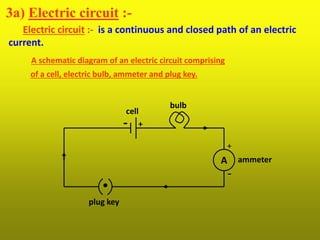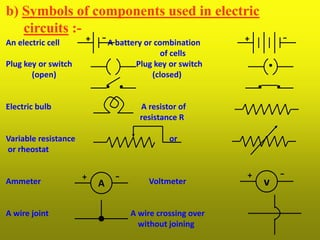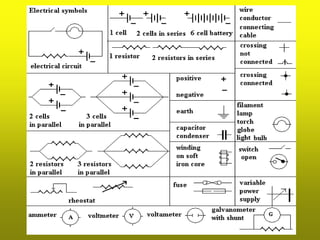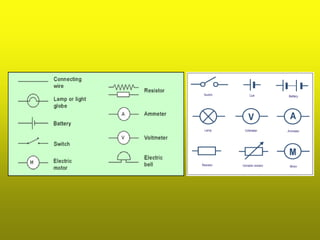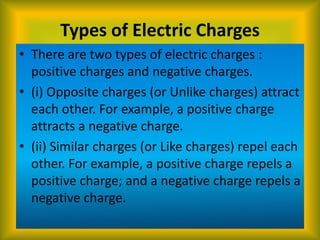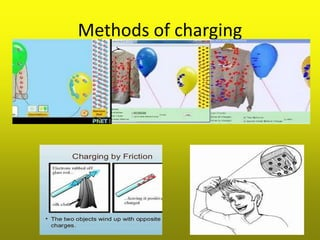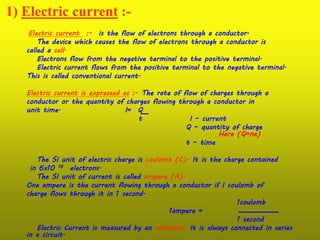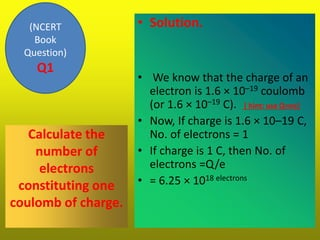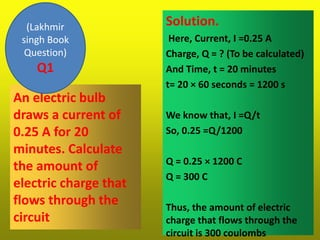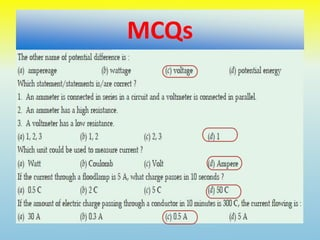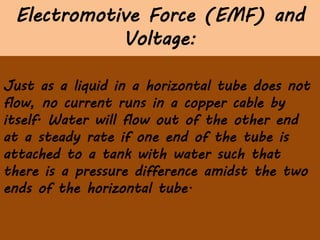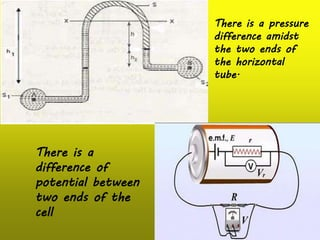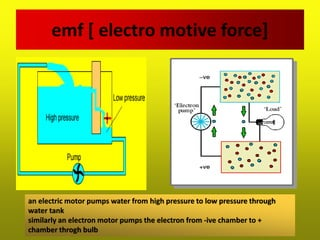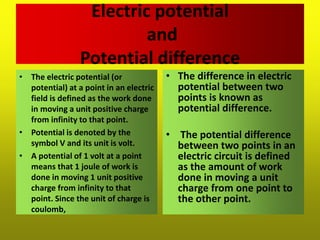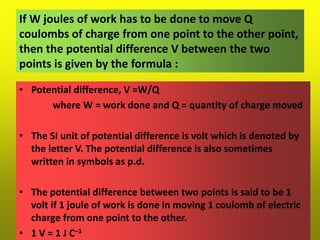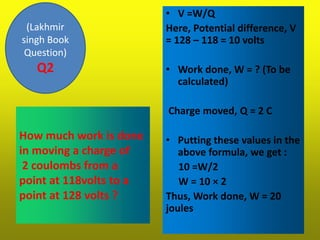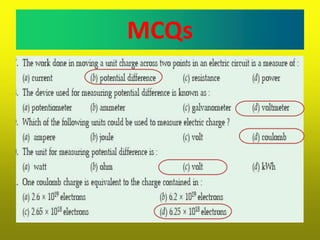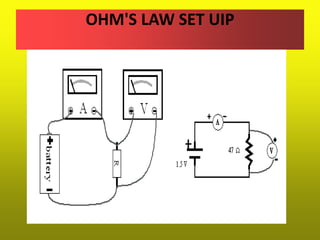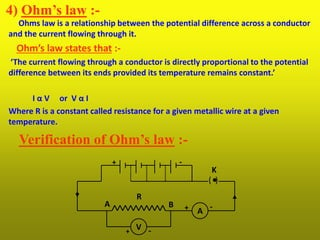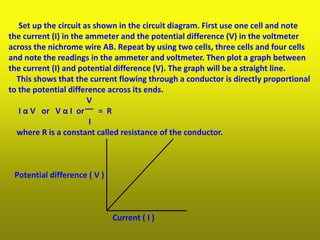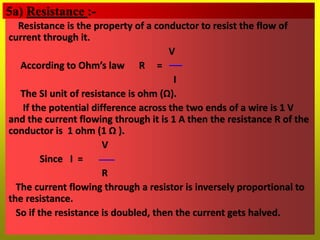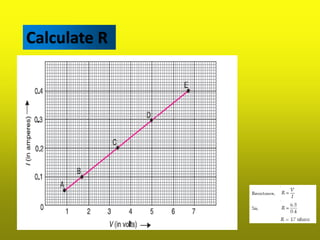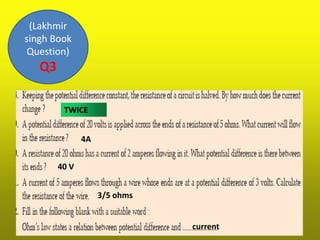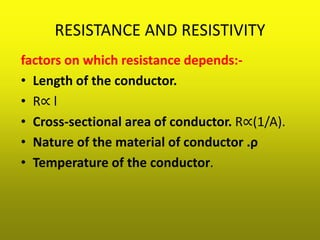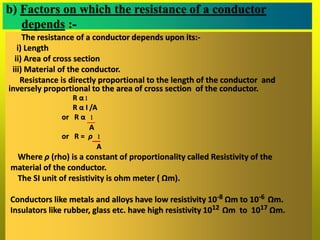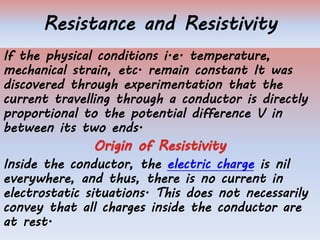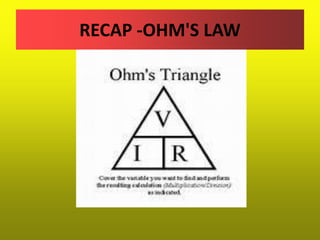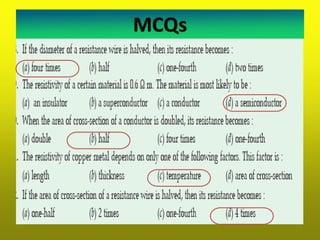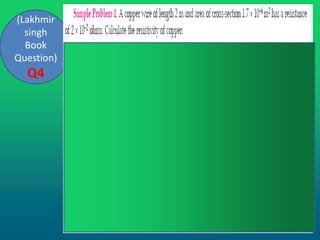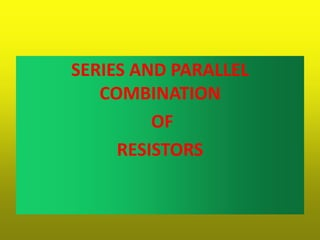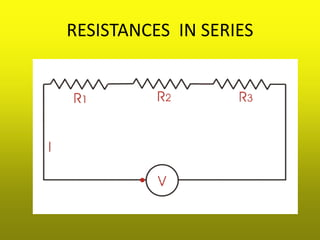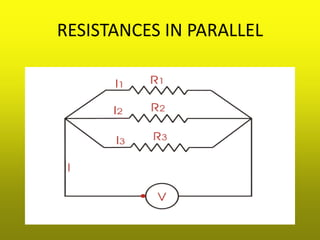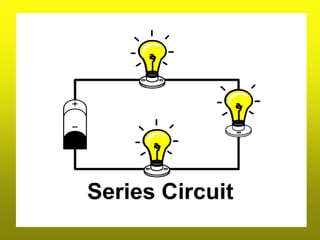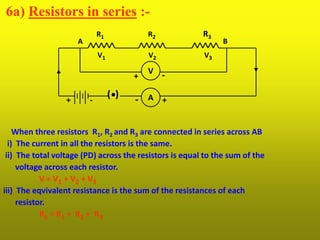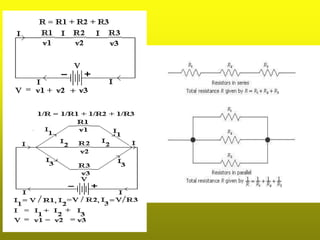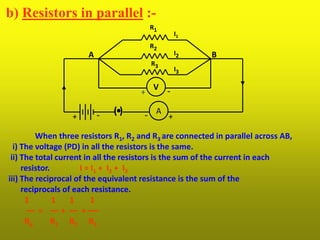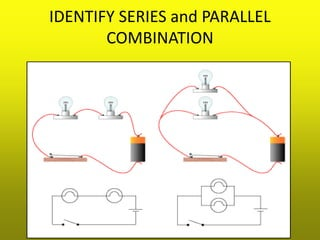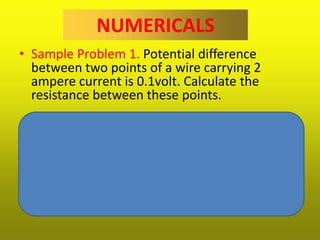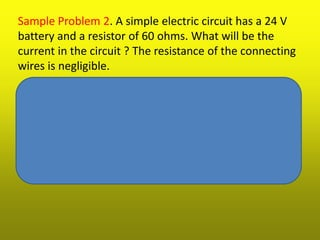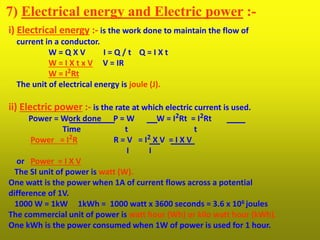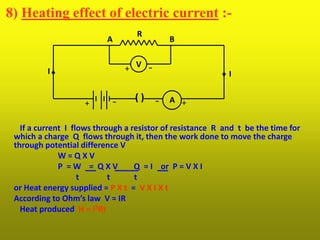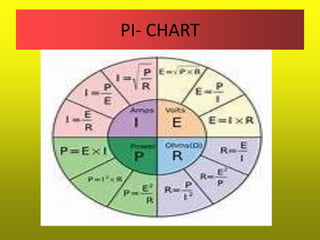1 von 64

### Current Electricity and Effects of Current

• 1. Electric current, potential difference and electric current. Ohm’s law; Resistance, Resistivity, Factors on which the resistance of a conductor depends. Series combination of resistors, parallel combination of resistors and its applications in daily life. Heating effect of electric current and its applications in daily life. Electric power, Interrelation between P, V, I and R Revised syllabus -CORONA PANDEMIC 2020 Effects of Current
• 2. What is Current Electricity? Any motion of charges from one section to another is current. When two bodies at different potentials are linked with a wire, free electrons stream from B to A, until both the objects reach the same potential, after which the current stops flowing. Until a potential difference is present throughout a conductor, current runs. The division of physics that deals with charges in motion is termed as current electricity.
• 10. current in a conductor
• 14. 3a) Electric circuit :- Electric circuit :- is a continuous and closed path of an electric current. A schematic diagram of an electric circuit comprising of a cell, electric bulb, ammeter and plug key. A + - +- bulb cell ammeter plug key
• 15. b) Symbols of components used in electric circuits :- An electric cell A battery or combination of cells Plug key or switch Plug key or switch (open) (closed) Electric bulb A resistor of resistance R Variable resistance or or rheostat Ammeter Voltmeter A wire joint A wire crossing over without joining A v+ - -+ + - + -
• 18. Types of Electric Charges • There are two types of electric charges : positive charges and negative charges. • (i) Opposite charges (or Unlike charges) attract each other. For example, a positive charge attracts a negative charge. • (ii) Similar charges (or Like charges) repel each other. For example, a positive charge repels a positive charge; and a negative charge repels a negative charge.
• 20. 1) Electric current :- Electric current :- is the flow of electrons through a conductor. The device which causes the flow of electrons through a conductor is called a cell. Electrons flow from the negative terminal to the positive terminal. Electric current flows from the positive terminal to the negative terminal. This is called conventional current. Electric current is expressed as :- The rate of flow of charges through a conductor or the quantity of charges flowing through a conductor in unit time. I= Q t I – current Q – quantity of charge Here (Q=ne) t – time The SI unit of electric charge is coulomb (C). It is the charge contained in 6x10 18 electrons. The SI unit of current is called ampere (A). One ampere is the current flowing through a conductor if I coulomb of charge flows through it in 1 second. 1coulomb 1ampere = 1 second Electric Current is measured by an ammeter. It is always connected in series in a circuit.
• 21. Calculate the number of electrons constituting one coulomb of charge. • Solution. • We know that the charge of an electron is 1.6 × 10–19 coulomb (or 1.6 × 10–19 C). [ hint: use Q=ne] • Now, If charge is 1.6 × 10–19 C, No. of electrons = 1 • If charge is 1 C, then No. of electrons =Q/e • = 6.25 × 1018 electrons (NCERT Book Question) Q1
• 22. An electric bulb draws a current of 0.25 A for 20 minutes. Calculate the amount of electric charge that flows through the circuit Solution. Here, Current, I =0.25 A Charge, Q = ? (To be calculated) And Time, t = 20 minutes t= 20 × 60 seconds = 1200 s We know that, I =Q/t So, 0.25 =Q/1200 Q = 0.25 × 1200 C Q = 300 C Thus, the amount of electric charge that flows through the circuit is 300 coulombs (Lakhmir singh Book Question) Q1
• 23. MCQs
• 24. Electromotive Force (EMF) and Voltage: Just as a liquid in a horizontal tube does not flow, no current runs in a copper cable by itself. Water will flow out of the other end at a steady rate if one end of the tube is attached to a tank with water such that there is a pressure difference amidst the two ends of the horizontal tube.
• 25. There is a pressure difference amidst the two ends of the horizontal tube. There is a difference of potential between two ends of the cell
• 26. emf [ electro motive force] an electric motor pumps water from high pressure to low pressure through water tank similarly an electron motor pumps the electron from -ive chamber to + chamber throgh bulb
• 27. Electric potential and Potential difference • The electric potential (or potential) at a point in an electric field is defined as the work done in moving a unit positive charge from infinity to that point. • Potential is denoted by the symbol V and its unit is volt. • A potential of 1 volt at a point means that 1 joule of work is done in moving 1 unit positive charge from infinity to that point. Since the unit of charge is coulomb, • The difference in electric potential between two points is known as potential difference. • The potential difference between two points in an electric circuit is defined as the amount of work done in moving a unit charge from one point to the other point.
• 28. If W joules of work has to be done to move Q coulombs of charge from one point to the other point, then the potential difference V between the two points is given by the formula : • Potential difference, V =W/Q where W = work done and Q = quantity of charge moved • The SI unit of potential difference is volt which is denoted by the letter V. The potential difference is also sometimes written in symbols as p.d. • The potential difference between two points is said to be 1 volt if 1 joule of work is done in moving 1 coulomb of electric charge from one point to the other. • 1 V = 1 J C–1
• 29. How much work is done in moving a charge of 2 coulombs from a point at 118volts to a point at 128 volts ? • V =W/Q Here, Potential difference, V = 128 – 118 = 10 volts • Work done, W = ? (To be calculated) Charge moved, Q = 2 C • Putting these values in the above formula, we get : 10 =W/2 W = 10 × 2 Thus, Work done, W = 20 joules (Lakhmir singh Book Question) Q2
• 30. MCQs
• 32. 4) Ohm’s law :- Ohms law is a relationship between the potential difference across a conductor and the current flowing through it. Ohm’s law states that :- ‘The current flowing through a conductor is directly proportional to the potential difference between its ends provided its temperature remains constant.’ I α V or V α I Where R is a constant called resistance for a given metallic wire at a given temperature. Verification of Ohm’s law :- V A + - + - + - R K A B ( )
• 34. Set up the circuit as shown in the circuit diagram. First use one cell and note the current (I) in the ammeter and the potential difference (V) in the voltmeter across the nichrome wire AB. Repeat by using two cells, three cells and four cells and note the readings in the ammeter and voltmeter. Then plot a graph between the current (I) and potential difference (V). The graph will be a straight line. This shows that the current flowing through a conductor is directly proportional to the potential difference across its ends. V I α V or V α I or = R I where R is a constant called resistance of the conductor. Potential difference ( V ) Current ( I )
• 35. 5a) Resistance :- Resistance is the property of a conductor to resist the flow of current through it. V According to Ohm’s law R = I The SI unit of resistance is ohm (Ω). If the potential difference across the two ends of a wire is 1 V and the current flowing through it is 1 A then the resistance R of the conductor is 1 ohm (1 Ω ). V Since I = R The current flowing through a resistor is inversely proportional to the resistance. So if the resistance is doubled, then the current gets halved.
• 38. RESISTANCE AND RESISTIVITY factors on which resistance depends:- • Length of the conductor. • R∝ l • Cross-sectional area of conductor. R∝(1/A). • Nature of the material of conductor .ρ • Temperature of the conductor.
• 39. b) Factors on which the resistance of a conductor depends :- The resistance of a conductor depends upon its:- i) Length ii) Area of cross section iii) Material of the conductor. Resistance is directly proportional to the length of the conductor and inversely proportional to the area of cross section of the conductor. R α l R α I /A or R α l A or R = ρ l A Where ρ (rho) is a constant of proportionality called Resistivity of the material of the conductor. The SI unit of resistivity is ohm meter ( Ωm). Conductors like metals and alloys have low resistivity 10-8 Ωm to 10-6 Ωm. Insulators like rubber, glass etc. have high resistivity 1012 Ωm to 1017 Ωm.
• 40. Resistance and Resistivity If the physical conditions i.e. temperature, mechanical strain, etc. remain constant It was discovered through experimentation that the current travelling through a conductor is directly proportional to the potential difference V in between its two ends. Inside the conductor, the electric charge is nil everywhere, and thus, there is no current in electrostatic situations. This does not necessarily convey that all charges inside the conductor are at rest.
• 42. MCQs
• 48. 6a) Resistors in series :- When three resistors R1, R2 and R3 are connected in series across AB i) The current in all the resistors is the same. ii) The total voltage (PD) across the resistors is equal to the sum of the voltage across each resistor. V = V1 + V2 + V3 iii) The eqvivalent resistance is the sum of the resistances of each resistor. RS = R1 + R2 + R3 ( ) A R1 R2 R3 + +-- V1 V2 V3 A B V + -
• 50. b) Resistors in parallel :- When three resistors R1, R2 and R3 are connected in parallel across AB, i) The voltage (PD) in all the resistors is the same. ii) The total current in all the resistors is the sum of the current in each resistor. I = I1 + I2 + I3 iii) The reciprocal of the equivalent resistance is the sum of the reciprocals of each resistance. 1 1 1 1 = + + Rp R1 R2 R3 R1 R3 R2 ( ) A I1 I2 I3 +-+ - A B V + -
• 51. IDENTIFY SERIES and PARALLEL COMBINATION
• 52. NUMERICALS • Sample Problem 1. Potential difference between two points of a wire carrying 2 ampere current is 0.1volt. Calculate the resistance between these points. • Solution- From Ohm’s law we have : R = V/I Resistance, R = 0.05 ohm
• 53. Sample Problem 2. A simple electric circuit has a 24 V battery and a resistor of 60 ohms. What will be the current in the circuit ? The resistance of the connecting wires is negligible. Solution - Using Ohm's law V=IR I = V/R I=24/60 I= 0.4 A
• 54. 7) Electrical energy and Electric power :- i) Electrical energy :- is the work done to maintain the flow of current in a conductor. W = Q X V I = Q / t Q = I X t W = I X t x V V = IR W = I2Rt The unit of electrical energy is joule (J). ii) Electric power :- is the rate at which electric current is used. Power = Work done P = W W = I2Rt = I2Rt Time t t Power = I2R R = V = I2 X V = I X V I I or Power = I X V The SI unit of power is watt (W). One watt is the power when 1A of current flows across a potential difference of 1V. 1000 W = 1kW 1kWh = 1000 watt x 3600 seconds = 3.6 x 106 joules The commercial unit of power is watt hour (Wh) or kilo watt hour (kWh). One kWh is the power consumed when 1W of power is used for 1 hour.
• 55. 8) Heating effect of electric current :- If a current I flows through a resistor of resistance R and t be the time for which a charge Q flows through it, then the work done to move the charge through potential difference V W = Q X V P = W = Q X V Q = I or P = V X I t t t or Heat energy supplied = P X t = V X I X t According to Ohm’s law V = IR Heat produced H = I2Rt ( ) A V R A B I I + - + - +-